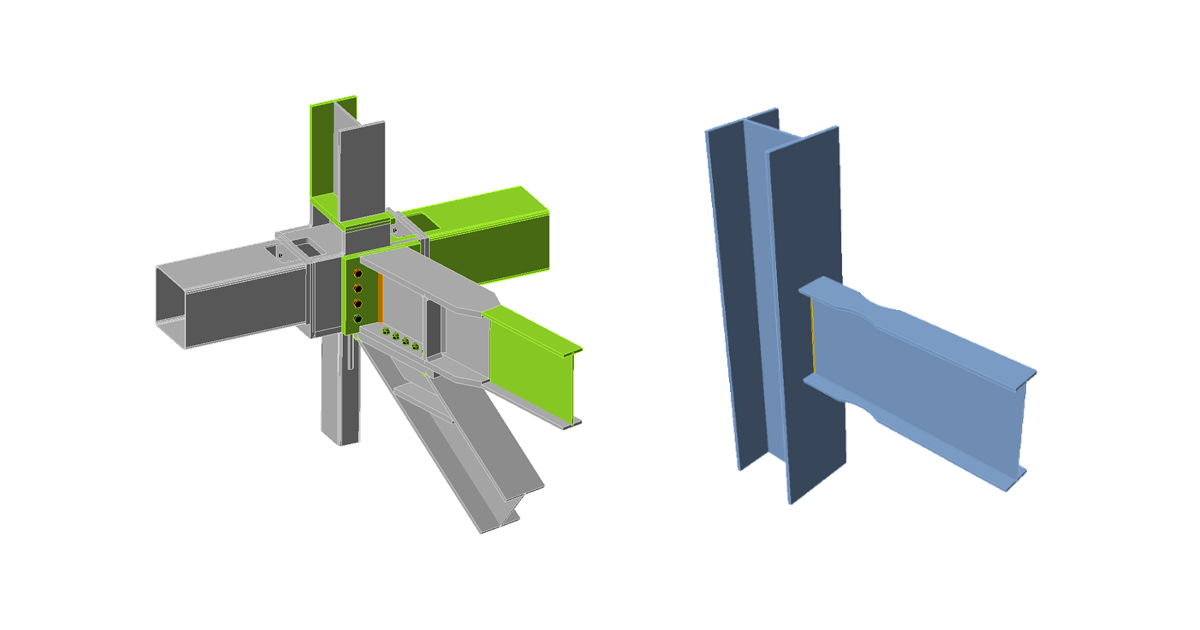### Choose language# Steel joint classification according to Canadian standards

$$Joints are classified according to joint stiffness to: Rigid – joints with insignificant change of original angles between members, Semirigid – joints which are assumed to have the capacity to furnish a dependable and known degree of flexural restraint, Simple – joints which do not develop bending moments. Canadian standard S14-16, Cl. 8.2 does not provide exact boundaries so the joints are classified according to the commentary in AISC 360-16, Cl. B3.4. Rigid – $$\frac{S_{j,ini} L_b}{E I_b} \ge 20$$ Semirigid – $$2 < \frac{S_{j,ini} L_b}{E I_b} < 20$$ Simple – $$\frac{S_{j,ini} L_b}{E I_b} \le 2$$ where: Sj,ini – initial stiffness of the joint; the joint stiffness is assumed linear up to the 2/3 of Mj,Rd Lb – theoretical length of the analyzed member E – Young's modulus of elasticity Ib – moment of inertia of the analyzed member Mj,Rd – joint design moment resistance$$